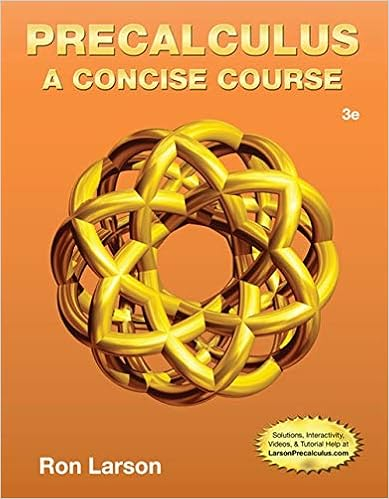# Marvelous motors is a small motor supply outlet that

• Test Prep
• 73
• 97% (463) 451 out of 463 people found this document helpful

This preview shows page 26 - 28 out of 73 pages.

##### We have textbook solutions for you!
The document you are viewing contains questions related to this textbook.The document you are viewing contains questions related to this textbook.
Chapter 2 / Exercise 77
Precalculus: A Concise Course
LarsonExpert Verified
12) Marvelous Motors is a small motor supply outlet that sells motors to companies that make various small motorized appliances. The fixed operating costs of the company are \$300,000 per year. The controlling shareholder, interested in product profitability and pricing, wants all costs allocated to the motors and wants to review the company status on a quarterly basis. The shareholder is trying to determine whether the costs should be allocated each quarter based on the 25% of the annual fixed operating costs (\$75,000) or by using an annual forecast budget to allocate the costs. The following information is provided for the operations of the company: ForecastActualSales for First Quarter5,0004,850Sales for Second Quarter8,0007,900Sales for Third Quarter8,0008,125Sales for Fourth Quarter3,0003,125Required:a.What amount of fixed operating costs are assigned to each motor by quarter when actual sales are used as the allocation base and \$75,000 is allocated?b.How much fixed cost is recovered each quarter under requirement a.?c.What amount of fixed operating costs are assigned to each motor by quarter when forecast sales are used as the allocation base and the rate is calculated annually as part of the budgetary process?d.How much fixed cost is recovered each quarter under requirement c.?e.Which method seems more appropriate in this case? Explain.Answer: a.Rate per unit using Actual Sales by Quarter:Q1 \$75,000 / 4,850 = \$15.46 per motorQ2 \$75,000 / 7,900 = \$ 9.49 per motorQ3 \$75,000 / 8,125 = \$ 9.23 per motorQ4 \$75,000 / 3,125 = \$24.00 per motorb.\$75,000 cost is recovered each quarter => \$300,000 cost recovered over the year.c.Quarterly Cost Recovery using Annual Forecast of Sales:Forecast Sales for the year = 5,000 + 8,000 + 8,000 + 3,000= 24,000Rate per motor = \$300,000 / 24,000 = \$12.50 per motord.Quarterly Cost Recovery using Annual Forecast of Sales as the allocation basis:Q1 4,850 × \$12.50 = \$ 60,625Q2 7,900 × \$12.50 = \$ 98,750Q3 8,125 × \$12.50 = \$101,563Q4 3,125 × \$12.50 = \$ 39,062 => \$300,000 cost recovered over the year
##### We have textbook solutions for you!
The document you are viewing contains questions related to this textbook.The document you are viewing contains questions related to this textbook.
Chapter 2 / Exercise 77
Precalculus: A Concise Course
LarsonExpert Verified
e.The budgeted rate based on an annualized forecast of sales is more appropriate to use.
Diff: 3Objective: 2AACSB: Application of knowledge
•••